# teaching machines

## Triangulation

May 10, 2021 by . Filed under public, slai-2021.

This post is part of a course on geometric modeling at the Summer Liberal Arts Institute for Computer Science held at Carleton College in 2021.

You’ve made a triangle. The logical next step is to make more complex shapes. You may not believe me, but more complex shapes are just made out of triangles. Any surface can be expressed as a quilt of triangles stitched together. The graphics card inside our computer, which is the hardware that renders 3D scenes, is really just a triangle processor. It knows one shape, and that’s it. Because of its narrow focus, hardware designers have been able to make it very fast.

Don’t believe me that complex shapes are just triangles? Consider these polygons: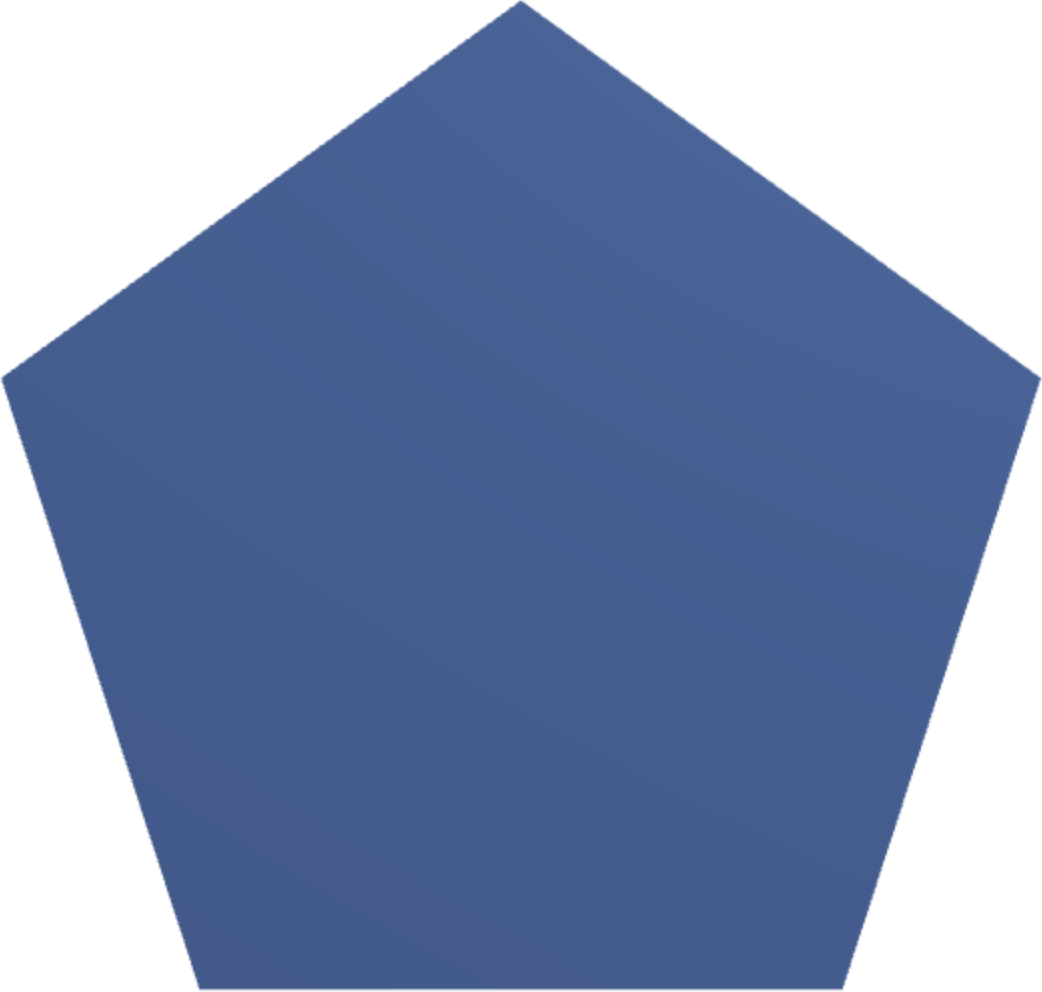They are just triangles: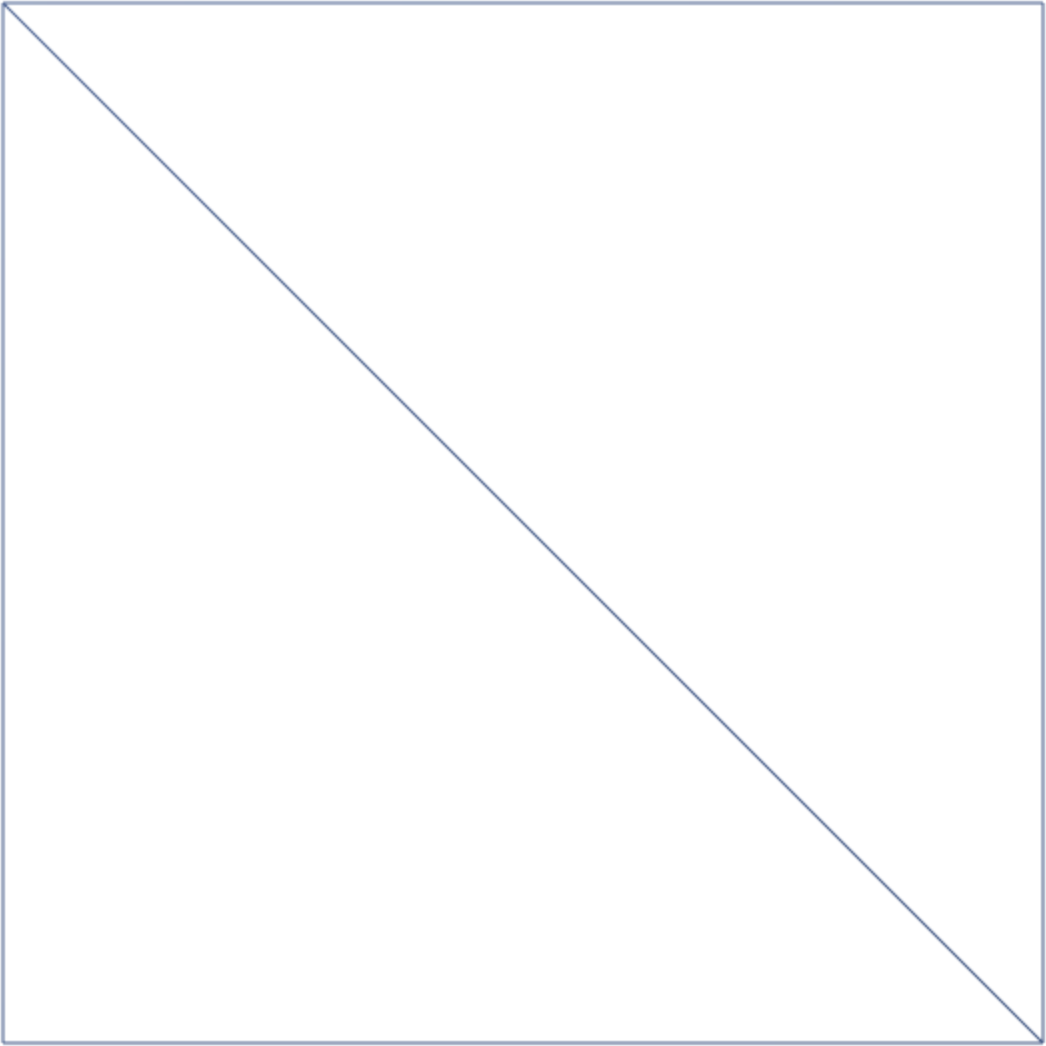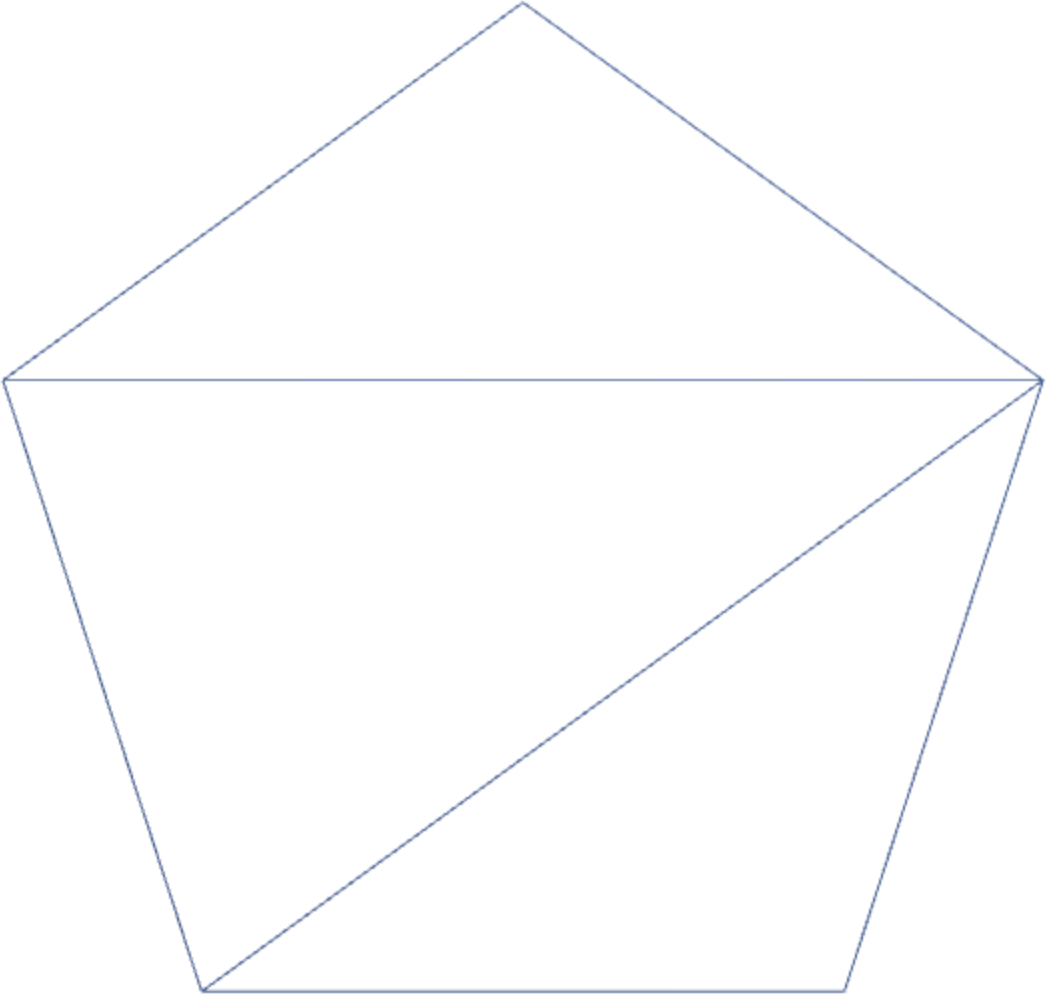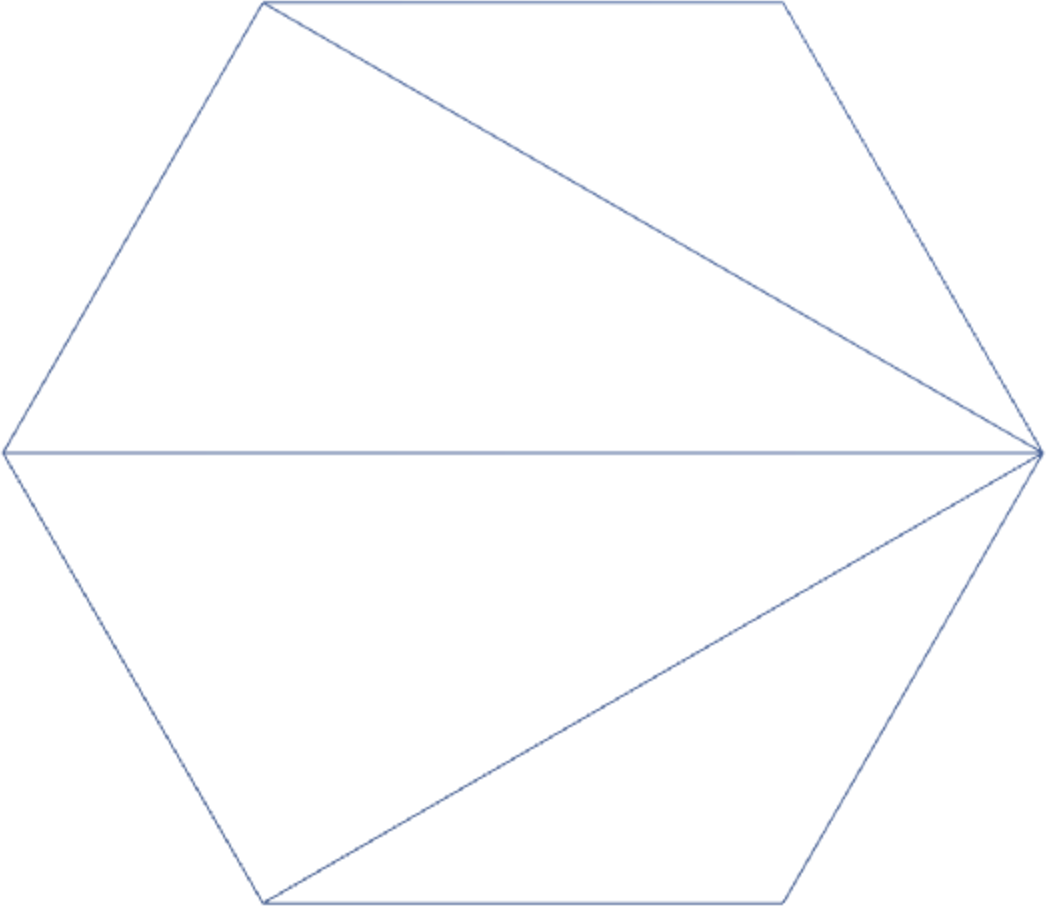Three examples are not an exhaustive proof. But it is true that any surface can be expressed as triangles. All of the shapes that you make in the next few days can be expressed with a structure like this:

const positions = [
// First triangle
vertex0.x, vertex0.y, vertex0.z,
vertex1.x, vertex1.y, vertex1.z,
vertex2.x, vertex2.y, vertex2.z,

// Second triangle
vertex3.x, vertex3.y, vertex3.z,
vertex4.x, vertex4.y, vertex4.z,
vertex5.x, vertex5.y, vertex5.z,

// Third triangle
vertex6.x, vertex6.y, vertex6.z,
vertex7.x, vertex7.y, vertex7.z,
vertex8.x, vertex8.y, vertex8.z,

// ...
];


In this code, each triangle is its own entity, independent of the others. This way of expressing shapes is simple but verbose. However, in the wireframe images above, you see that the triangles are not actually independent. They share vertices. For example, the square has just four vertices, not six. The two vertices on the diagonal are shared by both triangles. It’d be nice to not have to list those shared coordinates multiple times.

### Indexed Geometry

To eliminate the redundancy of shared vertices, we can express triangles as a triplet of integer indices instead of triplet of x-, y-, and z-coordinates. A square might have these four unique vertex positions, whose indices or positions with the array have been labeled in the comments:

const positions = [
-1, -1, 0, // Index 0
1, -1, 0, // Index 1
-1,  1, 0, // Index 2
1,  1, 0, // Index 3
];


A vertex’s index is implicit. The first three numbers form vertex 0, the second three form vertex 1, and so on.

You need a second array to group these indices together into triangles. If a vertex belongs to several triangles, its index will appear several times. Our second array looks like this:

const triangles = [
0, 1, 2,
1, 3, 2,
];


The first three indices form the first triangle, the second three form the second triangle, and so on.

To make THREE.js draw triangles based on the indices array rather than just positions, we must pass along the index list with this call:

geometry.setIndex(triangles);


Reload and you’ll see a square.

### Hexagon

Let’s get a little more practice with indexed geometry by constructing the hexagon. Here are its vertex positions:

const positions = [
1.0,  0.000, 0,
0.5,  0.866, 0,
-0.5,  0.866, 0,
-1.0,  0.000, 0,
-0.5, -0.866, 0,
0.5, -0.866, 0,
];


To help me think through this shape, I drew something like the following triangulation on graph paper: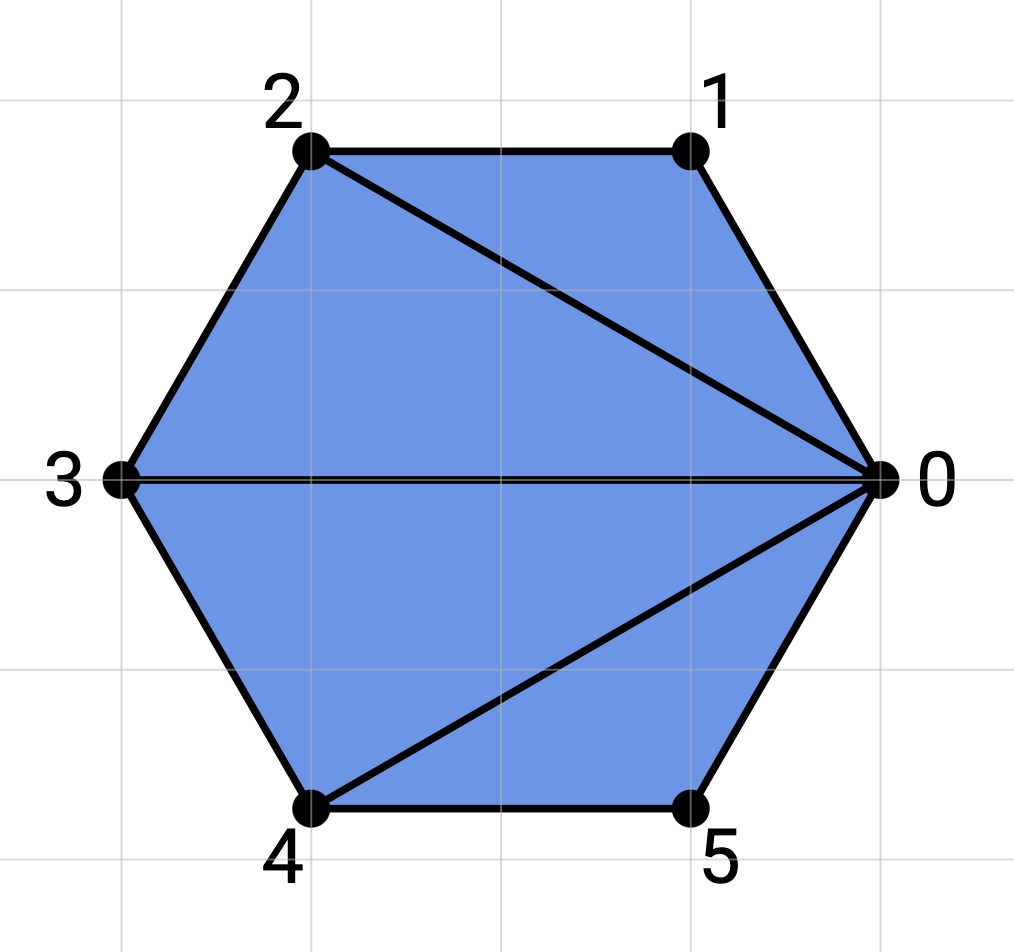Notice how the indices are labeled. By looking at the image, I can see how they group into triangles. Here’s our list of indices:

const triangles = [
0, 1, 2,
0, 2, 3,
0, 3, 4,
0, 4, 5,
];


You will be doing a lot of figuring out how to connect vertices together like this the next few days. The best advice I can give you this week is to always start your thinking on paper, away from the computer. Keep paper nearby.

### Challenge

Now it’s your turn to do some thinking and researching on your own. Adjust your renderer to produce a square donut that looks like this: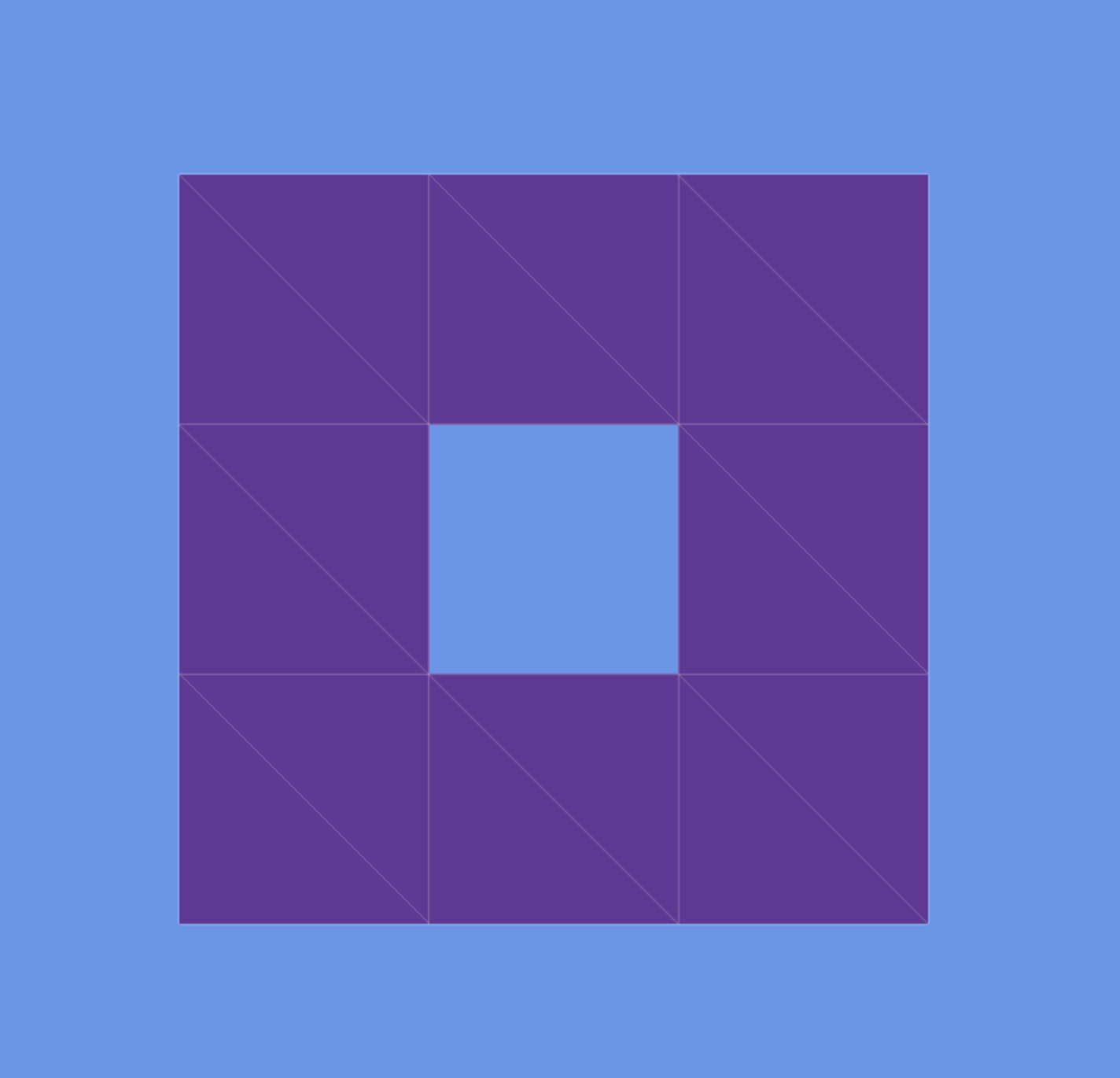Use indexed geometry. There are several ways to break this shape down into triangles. A wireframe overlay has been added to help you see how the triangles may be formed. There are other ways. The wireframe shows the way that will best prepare you for future exercises.

If you want to add your own wireframe overlay, consult the THREE.js documentation.